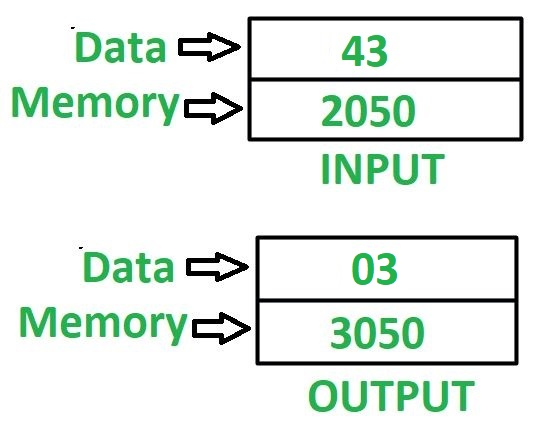# 8085 program to find minimum value of digit in the 8 bit number

• Last Updated : 28 May, 2018

Problem – Write an assembly language program in 8085 microprocessor to find minimum value of digit in the 8 bit number.

Example – Assume 8 bit number is stored at memory location 2050, and minimum value digit is stored at memory location 3050.Algorithm –

1. Load the content of memory location 2050 in accumulator A.
2. Move the content of A in register B.
3. Perform AND operation of content of A with 0F and store the result in A.
4. Move the content of A in register C.
5. Move the content of B in A.
6. Reverse the content of A by using RLC instruction 4 times.
7. Perform AND operation of content of A with 0F and store the result in A.
8. Compare the contents of A and C by help of CMP C instruction.
9. Check if carry flag is set then jump to memory location 2013 otherwise move the content of C in A. Go to memory location 2013.
10. Store the value of A in memory location 3050.

Note – CMP C instruction compares the value of A and C. If A>C then carry flag becomes reset otherwise set.

Program –

2000LDA 2050A <- M
2003MOV B, AB <- A
2004ANI 0FA <- A (AND) 0F
2006MOV C, AC <- A
2007MOV A, BA <- B
2008RLCRotate content of accumulator right by 1 bit without carry
2009RLCRotate content of accumulator right by 1 bit without carry
200ARLCRotate content of accumulator right by 1 bit without carry
200BRLCRotate content of accumulator right by 1 bit without carry
200CANI 0FA <- A (AND) 0F
200ECMP CA – C
200FJC 2013Jump if CY = 1
2012MOV A, CA <- C
2013STA 3050M <- A
2016HLTEND

Explanation – Registers A, B, C are used for general purpose.

1. LDA 2050: loads the contents of memory location 2050 in A.
2. MOV B, A: moves the content of A in B.
3. ANI 0F: perform AND operation between contents of A and value 0F.
4. MOV C, A: moves the content of A in C.
5. MOV A, B: moves the content of B in A.
6. RLC: shift the content of A left by 1 bit without carry. Use this instruction 4 times to reverse the content of A.
7. ANI 0F: perform AND operation between contents of A and value 0F.
8. CMP C: compare contents of A, C and update the value of carry flag accordingly.
9. JC 2013: jump to memory location 2013 if CY = 1.
10. MOV A, C: moves the content of C in A.
11. STA 3050: stores the content of A in memory location 3050.
12. HLT: stops executing the program and halts any further execution.
My Personal Notes arrow_drop_up